# Algebra II : Graphing Circular Inequalities

## Example Questions

2 Next →

### Example Question #11 : Graphing Circular Inequalities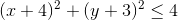Given the above circle inequality, does the center satisfy the equation?

Yes

No

Can't tell

Maybe

Yes

Explanation:

Recall the equation of circle: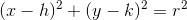where r is the radius and the center of the circle is at (h,k).

The center of the circle is (-4,-3), so plugging those values in for x and y yields the response that 0 is less than or equal to 4, which is a true statement, so the center does satisfy the inequality.

### Example Question #12 : Graphing Circular InequalitiesGiven the above circle inequality, is the shading on the graph inside or outside the circle?

Inside

Can't Tell

Both

Outside

Inside

Explanation:

Check the center of the circle to see if that point satisfies the inequality. When evaluating the function at the center (-4,-3), we see that it does satisfy the equation, so it can be in the shaded region of the graph. Therefore the shading is inside of the circle.

### Example Question #1041 : Algebra Ii

What is the-intercept of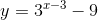There are no-intercepts of this function.Explanation:

The-intercepts of a function are the points where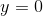. When we substitute this into our equation, we get: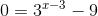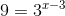Modifying the equation to get like bases get us,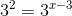Since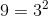.

Now we can set the exponents equal to eachother and solve for.

Thus,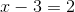Giving us our final solution:### Example Question #124 : Quadratic Functions

Which equation would produce this graph: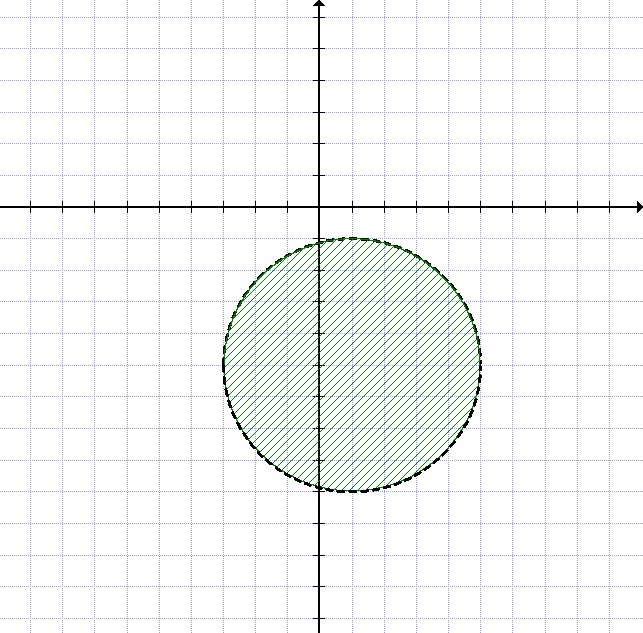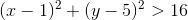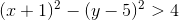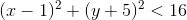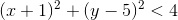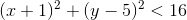Explanation:

The general equation of a circle iswhere the center is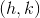and the radius is.

In this case, the center is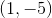and the radius is, so the equation for this circle is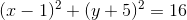.

The circle is shaded on the inside, which means that choosing any point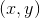and plugging it in for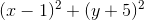would produce something less than.

Therefore, our answer is.

### Example Question #13 : Graphing Circular Inequalities

Which equation would match to this graph: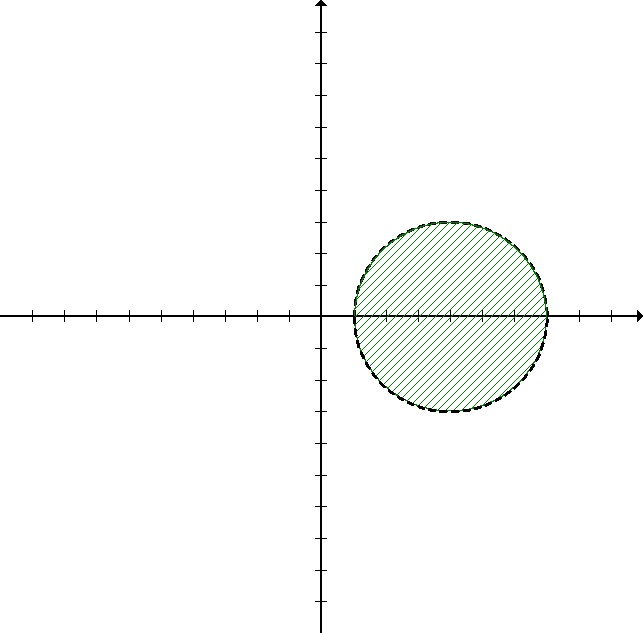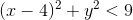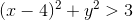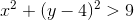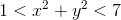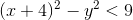Explanation:

The general equation for a circle iswhere the center isand its radius is.

In this case, the center is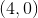and the radius is, so the equation for the circle is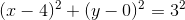.

We can simplify this equation to: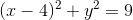.

The circle is shaded on the inside, which means that choosing any pointand plugging it in for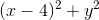would produce something less thanTherefore, our answer is.

### Example Question #21 : Quadratic Inequalities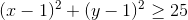Given the above circle inequality, which point satisfies the inequality?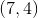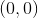Explanation:

The left side of the equation must be greater than or equal to 25 in order to satisfy the equation, so plugging in each of the values for x and y, we see that: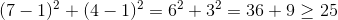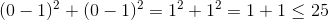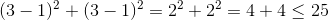The only point that satisfies the inequality is (7,4) since it yields an answer that is greater than or equal to 25.

2 Next →

### All Algebra II Resources